# Test: Comprehension Based Questions: Aldehydes, Ketones & Carboxylic Acids

## 19 Questions MCQ Test Chemistry 35 Years JEE Main & Advanced Past year Papers | Test: Comprehension Based Questions: Aldehydes, Ketones & Carboxylic Acids

Description
Attempt Test: Comprehension Based Questions: Aldehydes, Ketones & Carboxylic Acids | 19 questions in 40 minutes | Mock test for JEE preparation | Free important questions MCQ to study Chemistry 35 Years JEE Main & Advanced Past year Papers for JEE Exam | Download free PDF with solutions
QUESTION: 1

### PASSAGE -1 In the following reaction sequence, product I, J and L are formed. K represents a reagent.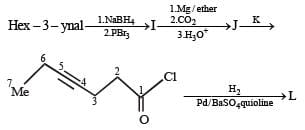Q.The structure of the product I is –

Solution: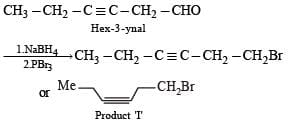Sodium borohydride reduces –CHO Selectively into –CH2O

QUESTION: 2

### In the following reaction sequence, product I, J and L are formed. K represents a reagent.Q. The structures of compound J and K, respectively, are

Solution: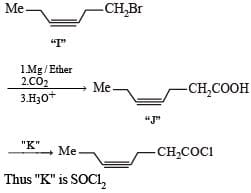QUESTION: 3

### In the following reaction sequence, product I, J and L are formed. K represents a reagent.Q. The structure of product L is

Solution: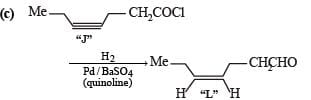It is Rosemmund reaction. Simultaneously the reagent H2-Pd also reduces carbon-carbon triple bond to double bond (syn -addition) giving cis product.

QUESTION: 4

PASSAGE-2

A carbonyl compound P,  which gives positive iodoform test, undergoes reaction with MeMgBr followed by dehydration to give an olefin Q. Ozonolysis of Q leads to a dicarbonyl compound R, which undergoes intramolecular aldol reaction to give predominantly S.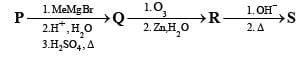Q. The structure of the carbonyl compound P is

Solution:

Iodoform test of compound P points out that P has – COCH3 group which shows that it may be either option (a) or (b) of Q. 16. Further since the dicarbonyl compound R has at least one a-H atom w.r.t to one of the carbonyl groups which is possible when R is produced from (b) of Q. 18; (a) option of Q. 16 will give dicarbonyl compound having two –CHO, none of which has a-H atom.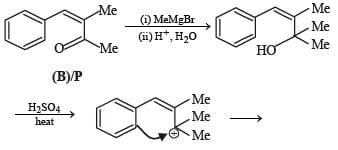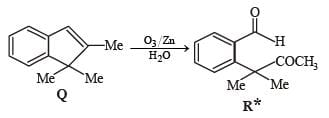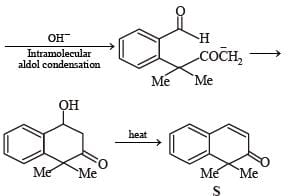* Structure of R would be R' when P is (A)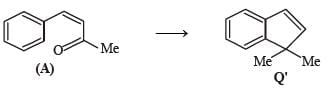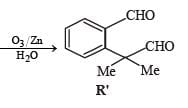QUESTION: 5

PASSAGE-2

A carbonyl compound P,  which gives positive iodoform test, undergoes reaction with MeMgBr followed by dehydration to give an olefin Q. Ozonolysis of Q leads to a dicarbonyl compound R, which undergoes intramolecular aldol reaction to give predominantly S.Q. The structures of the products Q and R, respectively, are

Solution:

Iodoform test of compound P points out that P has – COCH3 group which shows that it may be either option (a) or (b) of Q. 16. Further since the dicarbonyl compound R has at least one a-H atom w.r.t to one of the carbonyl groups which is possible when R is produced from (b) of Q. 18; (a) option of Q. 16 will give dicarbonyl compound having two –CHO, none of which has a-H atom.* Structure of R would be R' when P is (A)QUESTION: 6

PASSAGE-2

A carbonyl compound P,  which gives positive iodoform test, undergoes reaction with MeMgBr followed by dehydration to give an olefin Q. Ozonolysis of Q leads to a dicarbonyl compound R, which undergoes intramolecular aldol reaction to give predominantly S.Q. The structure of the product S is

Solution:

Iodoform test of compound P points out that P has – COCH3 group which shows that it may be either option (a) or (b) of Q. 16. Further since the dicarbonyl compound R has at least one a-H atom w.r.t to one of the carbonyl groups which is possible when R is produced from (b) of Q. 18; (a) option of Q. 16 will give dicarbonyl compound having two –CHO, none of which has a-H atom.* Structure of R would be R' when P is (A)QUESTION: 7

PASSAGE-3

Two aliphatic aldehydes P and Q react in the presence of aqueous K2CO3 to give compound R, which upon treatment with HCN provides compound S. On acidification and heating, S gives the product shown below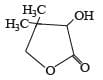Q. The compounds P and Q respectively are :

Solution:

Let us summarize the given facts of the problem.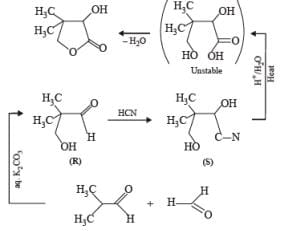(P & Q)

QUESTION: 8

PASSAGE-3

Two aliphatic aldehydes P and Q react in the presence of aqueous K2CO3 to give compound R, which upon treatment with HCN provides compound S. On acidification and heating, S gives the product shown below.Q. The compound R is :

Solution:

Let us summarize the given facts of the problem.(P & Q)

QUESTION: 9

PASSAGE-3

Two aliphatic aldehydes P and Q react in the presence of aqueous K2CO3 to give compound R, which upon treatment with HCN provides compound S. On acidification and heating, S gives the product shown below.

Q. The compound S is :

Solution:

Let us summarize the given facts of the problem.(P & Q)

QUESTION: 10

PASSAGE-4

In the following reaction sequence, the compound J is an intermediate.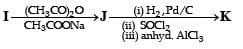J (C9H8O2) gives effervescence on treatment with NaHCO3 and a positive Baeyer’s test.

Q. The compound I is

Solution:

Reactions of compound J (C9H8O2) indicates that  it has C = C linkage and – COOH group. Thus, J can be written as C6H5CH = CH COOH. Since, J is unsaturated carboxylic acid and it is formed by the reactions of compound I with (CH3CO)2O and CH3COONa, compound I should be an aldehyde (recall Perkin reaction). Thus the whole series of reactions can be written as below.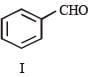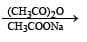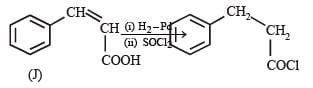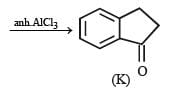QUESTION: 11

PASSAGE-4

In the following reaction sequence, the compound J is an intermediate.J (C9H8O2) gives effervescence on treatment with NaHCO3 and a positive Baeyer’s test.

Q. The compound K is

Solution:

Reactions of compound J (C9H8O2) indicates that  it has C = C linkage and – COOH group. Thus, J can be written as C6H5CH = CH COOH. Since, J is unsaturated carboxylic acid and it is formed by the reactions of compound I with (CH3CO)2O and CH3COONa, compound I should be an aldehyde (recall Perkin reaction). Thus the whole series of reactions can be written as below.QUESTION: 12

PASSAGE-5

P and Q are isomers of dicarboxylic acid C4H4O4. Both decolorize Br2/H2O. On heating, P forms the cyclic anhydride. Upon treatment with dilute alkaline KMnO4, P as well as Q could produce one or more than one from S, T and U.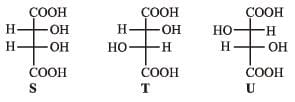Solution: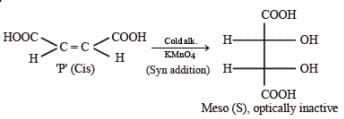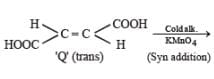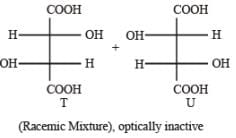QUESTION: 13

PASSAGE-5

P and Q are isomers of dicarboxylic acid C4H4O4. Both decolorize Br2/H2O. On heating, P forms the cyclic anhydride. Upon treatment with dilute alkaline KMnO4, P as well as Q could produce one or more than one from S, T and U.Q. In the following reaction sequences V and W are respectively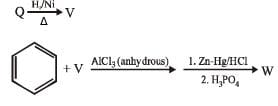Solution: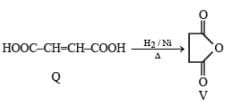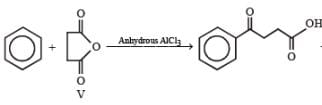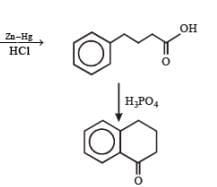QUESTION: 14

PASSAGE-6

In the following reactions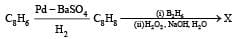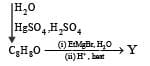Q. Compound X is

Solution: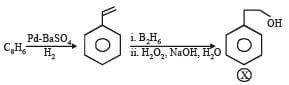QUESTION: 15

PASSAGE-6

In the following reactionsQ. The major compound Y is

Solution: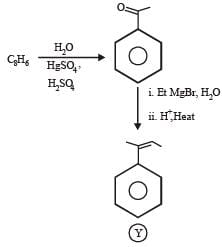QUESTION: 16

Each of  this question contains STATEMENT-1 (Assertion/ Statement ) and STATEMENT-2 (Reason/Explanation) and has 4 choices (a), (b), (c) and (d) out of which ONLY ONE is correct.

Q.

Statement-1 : Acetate ion is more basic than the methoxide ion.

Statement-2 : The acetate ion is resonance stabilized

Solution:

TIPS/Formulae :

Acetate ion is reasonance stabilized while methoxide ion is not.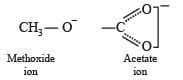Hence, acetate ion is less basic than methoxide ion.

QUESTION: 17

Statement-1 : Acetic acid does not undergo haloform reaction.

Statement-2 : Acetic acid has no alpha hydrogens.

Solution:

Haloform reaction is undergone only by ketones, CH3COOH has 3 α-hydrogens.

QUESTION: 18

Statement-1 : Dimethyl sulphide is commonly used for the reduction of an ozonide of an alkene to get the carbonyl compounds.

Statement-2 : It reduces the ozonide giving water soluble dimethyl sulphoxide and excess of it evaporates.

Solution:

TIPS/Formulae : Ozonide can be reduced by (CH3)2S to give carbonyl compounds and dimethyl sulphoxide.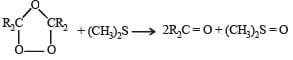QUESTION: 19

Statement-1 : p-Hydroxybenzoic acid has a lower boiling point than o-hydroxybenzoic acid.

Statement-2 : o-Hydroxybenzoic acid has intramolecular hydrogen bonding.

Solution:

p-Hydroxybenzoic acid has higher boiling point than o-hydroxybenzoic acid due to intermolecular hydrogen bonding. Thus, statement-1 is false. o-Hydroxybezoic acid shows intramolecular H-bonding thus, statement2 is true.Use Code STAYHOME200 and get INR 200 additional OFF Use Coupon Code